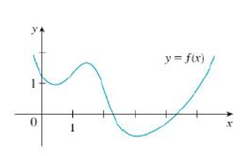Chapter 2.8, Problem 9E### Single Variable Calculus: Early Tr...

8th Edition
James Stewart
ISBN: 9781305270343

#### Solutions

Chapter
Section### Single Variable Calculus: Early Tr...

8th Edition
James Stewart
ISBN: 9781305270343
Textbook Problem

# Trace or copy the graph of the given function .f. (Assume that the axes have equal scales.) Then use the method of Example 1 to sketch the graph of f' below it.Example 1FIGURE 1FIGURE 2To determine

To sketch: The graph of f below the graph of f.

Explanation

From the graph, it is observed that the graph has a corner point at three points.

Let the three points be A, B and C. The derivative graph f is discontinuous at these points. That is, the derivative does not exist at these three points.

The graph from point A to B has negative slope which implies that the derivative graph must have negative value in this section. Since the function is linear in this part,

the derivative graph will be horizontal line.

The graph from point B to C has positive slope which implies that the derivative graph must have positive value in this section. Since the function is linear in this part,

the derivative graph will be horizontal line.

The graph from point C to D has negative slope which implies that the derivative graph must have a negative functional value

### Still sussing out bartleby?

Check out a sample textbook solution.

See a sample solution

#### The Solution to Your Study Problems

Bartleby provides explanations to thousands of textbook problems written by our experts, many with advanced degrees!

Get Started

#### Place the following set of n = 20 scores in a frequency distribution table.

Essentials of Statistics for The Behavioral Sciences (MindTap Course List)

#### In Exercises 13-20, sketch a set of coordinate axes and plot each point. 17. (8,72)

Applied Calculus for the Managerial, Life, and Social Sciences: A Brief Approach

#### For

Study Guide for Stewart's Multivariable Calculus, 8th

#### The third partial sum of is:

Study Guide for Stewart's Single Variable Calculus: Early Transcendentals, 8th

#### Graph each equation. 3x5y=3(x5)

College Algebra (MindTap Course List)